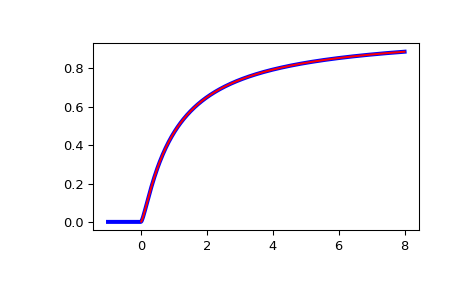# scipy.special.ncfdtr¶

scipy.special.ncfdtr(dfn, dfd, nc, f) = <ufunc 'ncfdtr'>

Cumulative distribution function of the non-central F distribution.

The non-central F describes the distribution of,

$Z = \frac{X/d_n}{Y/d_d}$

where $$X$$ and $$Y$$ are independently distributed, with $$X$$ distributed non-central $$\chi^2$$ with noncentrality parameter nc and $$d_n$$ degrees of freedom, and $$Y$$ distributed $$\chi^2$$ with $$d_d$$ degrees of freedom.

Parameters
dfnarray_like

Degrees of freedom of the numerator sum of squares. Range (0, inf).

dfdarray_like

Degrees of freedom of the denominator sum of squares. Range (0, inf).

ncarray_like

Noncentrality parameter. Should be in range (0, 1e4).

farray_like

Quantiles, i.e. the upper limit of integration.

Returns
cdffloat or ndarray

The calculated CDF. If all inputs are scalar, the return will be a float. Otherwise it will be an array.

ncfdtri

Quantile function; inverse of ncfdtr with respect to f.

ncfdtridfd

Inverse of ncfdtr with respect to dfd.

ncfdtridfn

Inverse of ncfdtr with respect to dfn.

ncfdtrinc

Inverse of ncfdtr with respect to nc.

Notes

Wrapper for the CDFLIB  Fortran routine cdffnc.

The cumulative distribution function is computed using Formula 26.6.20 of :

$F(d_n, d_d, n_c, f) = \sum_{j=0}^\infty e^{-n_c/2} \frac{(n_c/2)^j}{j!} I_{x}(\frac{d_n}{2} + j, \frac{d_d}{2}),$

where $$I$$ is the regularized incomplete beta function, and $$x = f d_n/(f d_n + d_d)$$.

The computation time required for this routine is proportional to the noncentrality parameter nc. Very large values of this parameter can consume immense computer resources. This is why the search range is bounded by 10,000.

References

1(1,2)

Barry Brown, James Lovato, and Kathy Russell, CDFLIB: Library of Fortran Routines for Cumulative Distribution Functions, Inverses, and Other Parameters.

2(1,2)

Milton Abramowitz and Irene A. Stegun, eds. Handbook of Mathematical Functions with Formulas, Graphs, and Mathematical Tables. New York: Dover, 1972.

Examples

>>> from scipy import special
>>> from scipy import stats
>>> import matplotlib.pyplot as plt


Plot the CDF of the non-central F distribution, for nc=0. Compare with the F-distribution from scipy.stats:

>>> x = np.linspace(-1, 8, num=500)
>>> dfn = 3
>>> dfd = 2
>>> ncf_stats = stats.f.cdf(x, dfn, dfd)
>>> ncf_special = special.ncfdtr(dfn, dfd, 0, x)

>>> fig = plt.figure()